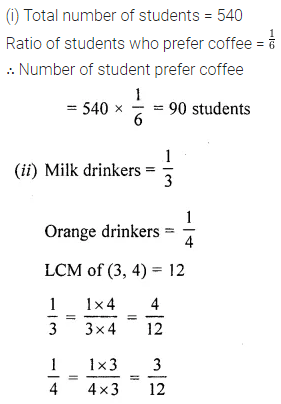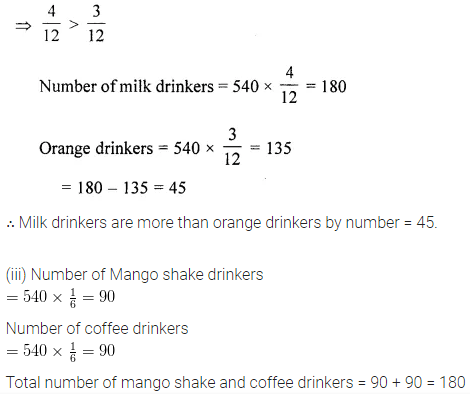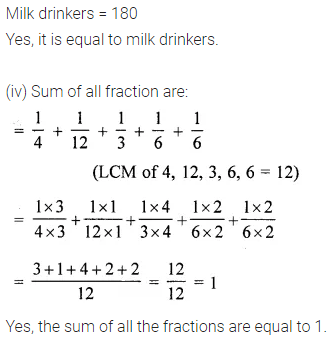# ML Aggarwal Class 6 Solutions for ICSE Maths Chapter 6 Fractions Objective Type Questions

## ML Aggarwal Class 6 Solutions for ICSE Maths Chapter 6 Fractions Objective Type Questions

Mental Maths
Question 1.
Fill in the blanks:
(i) A fraction is a number which represent a ………… of whole.
(ii) A proper fraction lies between 0 and …………
(iii) A mixed fraction can be converted into ………… fraction.
(iv) Fractions having different denominations are called …………
(v) In two like fractions, the fraction having smaller numerator is …………
(vi) $$\frac{144}{180}$$ reduced to simplest form is …………..
(vii) $$7 \frac{2}{5}$$ + ………… = 12
(viii) $$\frac{42}{56}=\frac{6}{\dots . .}$$
Solution: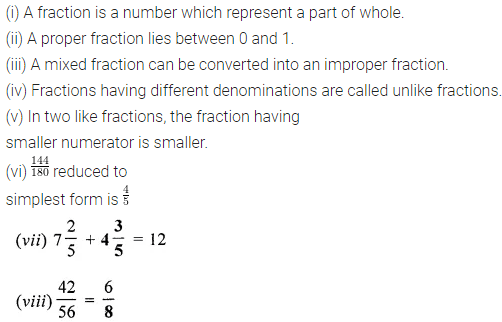Question 2.
State whether the following statements are true (T) or false (F):
(i) Two fractions with same numerator are called like fractions.
(ii) A fraction in which the numerator is greater than is denominator is called an improper fraction.
(iii) Every improper fract on can be converted into a mixed fraction.
(iv) Every fraction can be represented by a point on a number line.
(v) In two unlike fractions with same numerator, the fraction having greater denominator is greater.
(vi) $$\frac{1}{2}, \frac{1}{3} \text { and } \frac{1}{4}$$ are like fractions.
(vii) $$5-1 \frac{3}{4}=4 \frac{1}{4}$$
Solution:Multiple Choice Questions
Choose the correct answer from the given four options (3 to 17):
Question 3.
In the given figure, the shaded part is represented by the fraction :Solution: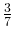Question 4.
In the given figure, the shaded region is represented by the fraction :Solution:Question 5.
The two consecutive integers between which the fraction $$\frac{5}{7}$$ lies are
(a) 5 and 7
(b) 5 and 6
(c) 6 and 7
(d) 0 and 1
Solution: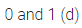Question 6.
Which of the following pairs of fractions are not equivalent?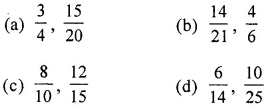Solution: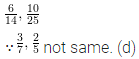Question 7.
The fraction equivalent to $$\frac{45}{81}$$ is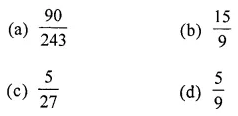Solution: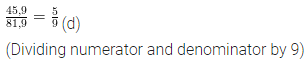Question 8.
The fraction which is not equal to $$\frac{4}{5}$$ is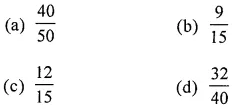Solution:Question 9.
Which of the following fractions is not in the lowest form?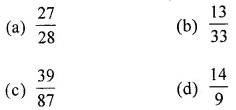Solution: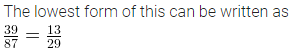Question 10.
A pair of like fraction is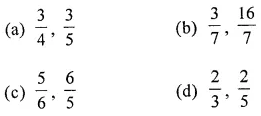Solution: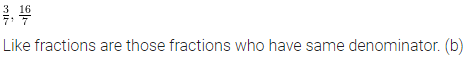Question 11.
Which of the following fractions is the greatest?Solution: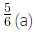Question 12.
Which of the following fractions is the smallest?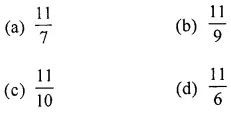Solution: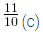Question 13.
Which of the following is a false statement?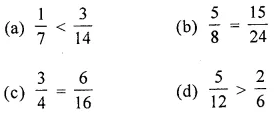Solution:Question 14.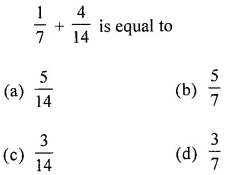Solution: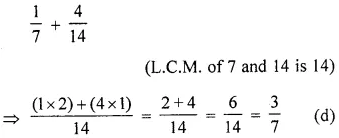Question 15.Solution: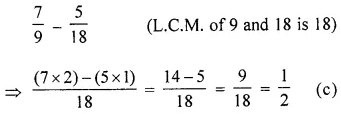Question 16.
Anshul eats $$\frac{4}{7}$$ of a pizza. The fraction of the pizza left isSolution:Question 17.
The fraction whose numerator is the smallest odd prime number and denominator is the smallest composite number is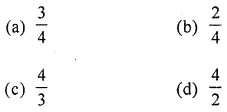Solution:Higher Order Thinking Skills (Hots)
Question 1.
Write all proper fractions whose sum of numerator and denominator is 12.
Solution: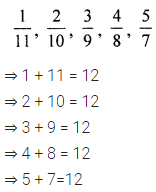Question 2.
The given figure represents the preferences of the students during breakfast in a hostel mess. If the total number of students in the mess is 540, then with reference to the given figure, answer the following questions: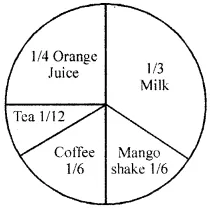(i) What is the number of students who prefer coffee?
(ii) Whose number is greater, milk drinkers or
orange juice drinkers and by what number?
(iii) What is the total number of students who drink
mango shake or coffee? Is it equal to milk drinker?
(iv) Is the sum of all fractions in the given figure equal to 1 ?
Solution: